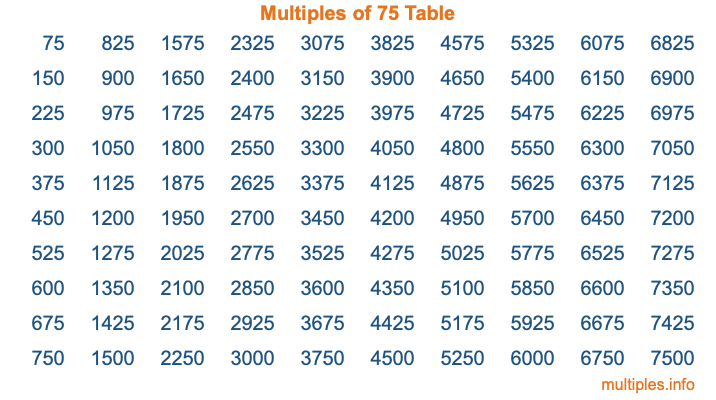Multiples of 75Welcome to the Multiples of 75 page. Here we will first teach you everything you will ever need to know about the multiples of 75, and then give you a study guide summary of everything we taught you to make sure you remember it all. Use this page to look up facts and learn information about the multiples of 75. This page will make you a multiples of seventy-five expert!

Definition of Multiples of 75
Multiples of 75 are all the numbers that when divided by 75 equal an integer. Each of the multiples of 75 are called a multiple. A multiple of 75 is created by multiplying 75 by an integer.

Therefore, to create a list of multiples of 75, you start with 1 multiplied by 75, then 2 multiplied by 75, then 3 multiplied by 75, and so on for as long as you want. Thus, the list of the first five multiples of 75 is 75, 150, 225, 300, and 375. To see a larger list of multiples of 75, see the printable image of Multiples of 75 further down on this page. We also have a category where you can choose any nth multiple of 75.

Multiples of 75 Checker
The Multiples of 75 Checker below checks to see if any number of your choice is a multiple of 75. In other words, it checks to see if there is any number (integer) that when multiplied by 75 will equal your number. To do that, we divide your number by 75. If the the quotient is an integer, then your number is a multiple of 75.

Is  a multiple of 75?

Least Common Multiple of 75 and ...
A Least Common Multiple (LCM) is the lowest multiple that two or more numbers have in common. This is also called the smallest common multiple or lowest common multiple and is useful to know when you are adding our subtracting fractions. Enter one or more numbers below (75 is already entered) to find the LCM.

Check out our LCM Calculator if you need more details about the Least Common Multiple or if you need the LCM for different numbers for adding and subtraction fractions.

nth Multiple of 75
As we stated above, 75 is the first multiple of 75, 150 is the second multiple of 75, 225 is the third multiple of 75, and so on. Enter a number below to find the nth multiple of 75.

th multiple of 75

Multiples of 75 vs Factors of 75
75 is a multiple of 75 and a factor of 75, but that is where the similarities end. All postive multiples of 75 are 75 or greater than 75. All positive factors of 75 are 75 or less than 75.

Below is the beginning list of multiples of 75 and the factors of 75 so you can compare:

Multiples of 75: 75, 150, 225, 300, 375, etc.

Factors of 75: 1, 3, 5, 15, 25, 75

As you can see, the multiples of 75 are all the numbers that you can divide by 75 to get a whole number. The factors of 75, on the other hand, are all the whole numbers that you can multiply by another whole number to get 75.

It's also interesting to note that if a number (x) is a factor of 75, then 75 will also be a multiple of that number (x).

Multiples of 75 vs Divisors of 75
The divisors of 75 are all the integers that 75 can be divided by evenly. Below is a list of the divisors of 75.

Divisors of 75: 1, 3, 5, 15, 25, 75

The interesting thing to note here is that if you take any multiple of 75 and divide it by a divisor of 75, you will see that the quotient is an integer.

Multiples of 75 Table
Below is an image of the first 100 multiples of 75 in a table. The table is in chronological order, column by column. The first column has the first ten multiples of 75, the second column has the next ten multiples of 75, and so on.The Multiples of 75 Table is also referred to as the 75 Times Table or Times Table of 75. You are welcome to print out our table for your studies.

Negative Multiples of 75
Although not often discussed or needed in math, it is worth mentioning that you can make a list of negative multiples of 75 by multiplying 75 by -1, then by -2, then by -3, and so on, to get the following list of negative multiples of 75:

-75, -150, -225, -300, -375, etc.

Multiples of 75 Summary
Below is a summary of important Multiples of 75 facts that we have discussed on this page. To retain the knowledge on this page, we recommend that you read through the summary and explain to yourself or a study partner why they hold true.

There are an infinite number of multiples of 75.

A multiple of 75 divided by 75 will equal a whole number.

75 divided by a factor of 75 equals a divisor of 75.

The nth multiple of 75 is n times 75.

The largest factor of 75 is equal to the first positive multiple of 75.

75 is a multiple of every factor of 75.

75 is a multiple of 75.

A multiple of 75 divided by a divisor of 75 equals an integer.

75 divided by a divisor of 75 equals a factor of 75.

Any integer times 75 will equal a multiple of 75.

Multiples of a Number
Here you can get the multiples of another number, all with the same attention to detail as we did for multiples of 75 on this page.

Multiples of
Multiples of 76
Did you find our page about multiples of seventy-five educational? Do you want more knowledge? Check out the multiples of the next number on our list!